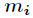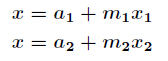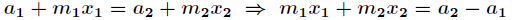# 中国剩余定理学习笔记

### 题意：

人自出生起就有体力，情感和智力三个生理周期，分别为23，28和33天。一个周期内有一天为峰值，在这一天，人在对应的方面（体力，情感或智力）表现最好。通常这三个周期的峰值不会是同一天。现在给出三个日期，分别对应于体力，情感，智力出现峰值的日期。然后再给出一个起始日期，要求从这一天开始，算出最少再过多少天后三个峰值同时出现。

### 分析：

首先我们要知道，任意两个峰值之间一定相距整数倍的周期。假设一年的第N天达到峰值，则下次达到峰值的时间为${N+Tk}$(${T}$是周期，${k}$是任意正整数)。所以，三个峰值同时出现的那一天${(S)}$应满足$${S = N_1 + T_1*k_1 = N_2 + T_2*k_2 = N_3 + T_3*k_3}$$

${N1,N2,N3}$分别为为体力，情感，智力出现峰值的日期， ${T1，T2,T3}$分别为体力，情感，智力周期。 我们需要求出${k1,k2,k3}$三个非负整数使上面的等式成立。

想直接求出${k1,k2,k3}$貌似很难，但是我们的目的是求出${S}$， 可以考虑从结果逆推。根据上面的等式，${S}$满足三个要求：除以${T_1}$余数为${N_1}$，除以${T_2}$余数为${N_2}$，除以${T_3}$余数为${N_3}$。这样我们就把问题转化为求一个最小数，该数除以${T_1}$余${N_1}$，除以${T_2}$余${N_2}$，除以${T_3}$余${N_3}$。这就是著名的中国剩余定理，我们的老祖宗在几千年前已经对这个问题想出了一个精妙的解法。依据此解法的算法，时间复杂度可达到${O(1)}$。

## 中国剩余定理

在《孙子算经》中有这样一个问题：“今有物不知其数，三三数之剩二（除以3余2），五五数之剩三（除以5余3），七七数之剩二（除以7余2），问物几何？”这个问题称为“孙子问题”，该问题的一般解法国际上称为“中国剩余定理”。具体解法分三步：

1. 找出三个数：从3和5的公倍数中找出被7除余1的最小数15，从3和7的公倍数中找出被5除余1 的最小数21，最后从5和7的公倍数中找出除3余1的最小数70。
2. 用15乘以2（2为最终结果除以7的余数），用21乘以3（3为最终结果除以5的余数），同理，用70乘以2（2为最终结果除以3的余数），然后把三个乘积相加${15*2+21*3+70*2}$得到和233。
3. 用233除以3，5，7三个数的最小公倍数105，得到余数23，即${ 233\%105=23 }$。这个余数23就是符合条件的最小数。

就这么简单。我们在感叹神奇的同时不禁想知道古人是如何想到这个方法的，有什么基本的数学依据吗？

我们将“孙子问题”拆分成几个简单的小问题，从零开始，试图揣测古人是如何推导出这个解法的。

首先，我们假设${n_1}$是满足除以3余2的一个数，比如2，5，8等等，也就是满足${3*k+2（k>=0）}$的一个任意数。同样，我们假设${n_2}$是满足除以5余3的一个数，${n_3}$是满足除以7余2的一个数。

有了前面的假设，我们先从${n_1}$这个角度出发，已知${n_1}$满足除以3余2，能不能使得${n_1+n_2}$的和仍然满足除以3余2？进而使得${n_1+n_2+n_3}$的和仍然满足除以3余2？

这就牵涉到一个最基本数学定理，如果有${ a \% b=c }$，则有${ (a+k*b) \% b=c(k为非零整数) }$，换句话说，如果一个除法运算的余数为${c}$，那么被除数与${k}$倍的除数相加（或相减）的和（差）再与除数相除，余数不变。这个是很好证明的。

以此定理为依据，如果${n_2}$是3的倍数，${n_1+n_2}$就依然满足除以3余2。同理，如果${n_3}$也是3的倍数，那么${n_1+n_2+n_3}$的和就满足除以3余2。这是从${n_1}$的角度考虑的，再从${n_2}$，${n_3}$的角度出发，我们可推导出以下三点：

1. 为使${n_1+n_2+n_3}$的和满足除以3余2，${n_2}$和${n_3}$必须是3的倍数。
2. 为使${n_1+n_2+n_3}$的和满足除以5余3，${n_1}$和${n_3}$必须是5的倍数。
3. 为使${n_1+n_2+n_3}$的和满足除以7余2，${n_1}$和${n_2}$必须是7的倍数。

因此，为使${n_1+n_2+n_3}$的和作为“孙子问题”的一个最终解，需满足：

1. ${n_1}$除以3余2，且是5和7的公倍数。
2. ${n_2}$除以5余3，且是3和7的公倍数。
3. ${n_3}$除以7余2，且是3和5的公倍数。

所以，孙子问题解法的本质是从5和7的公倍数中找一个除以3余2的数${n_1}$，从3和7的公倍数中找一个除以5余3的数${n_2}$，从3和5的公倍数中找一个除以7余2的数${n_3}$，再将三个数相加得到解。在求${n_1}$，${n_2}$，${n_3}$时又用了一个小技巧，以${n_1}$为例，并非从5和7的公倍数中直接找一个除以3余2的数，而是先找一个除以3余1的数，再乘以2。也就是先求出5和7的公倍数模3下的逆元，再用逆元去乘余数

这里又有一个数学公式，如果${a \% b=c}$，那么${(a*k) \% b=a \% b+a \% b+…+a \% b=c+c+…+c=k*c(k>0)}$,也就是说，如果一个除法的余数为${c}$，那么被除数的${k}$倍与除数相除的余数为${k*c}$。展开式中已证明。

最后，我们还要清楚一点，${n_1+n_2+n_3}$只是问题的一个解，并不是最小的解。如何得到最小解？我们只需要从中最大限度的减掉掉3，5，7的公倍数105即可。道理就是前面讲过的定理“如果${a \%b=c}$，则有${(a-k*b) \% b=c}$”。所以${（n_1+n_2+n_3）\% 105}$就是最终的最小解。

这样一来就得到了中国剩余定理的公式：## 中国剩余定理扩展——求解模数不互质情况下的线性方程组：

普通的中国剩余定理要求所有的互素，那么如果不互素呢，怎么求解同余方程组？

这种情况就采用两两合并的思想，假设要合并如下两个方程：那么得到：我们需要求出一个最小的${x}$使它满足：那么${x_1}$和${x_2}$就要尽可能的小，于是我们用扩展欧几里得算法求出${x_1}$的最小正整数解，将它代回${a_1+m_1x_1}$，得到${x}$的一个特解${x'}$，当然也是最小正整数解。

所以${x}$的通解一定是${x'}$加上${lcm(m1,m2)*k}$，这样才能保证${x}$模${m_1}$和${m_2}$的余数是${a_1}$和${a_2}$。由此，我们把这个${x'}$当做新的方程的余数，把${lcm(m1,m2)}$当做新的方程的模数。（这一段是关键

合并完成：http://www.cnblogs.com/walker01/archive/2010/01/23/1654880.html

http://blog.csdn.net/acdreamers/article/details/8050018

posted @ 2016-09-28 22:17  MashiroSky  阅读(36107)  评论(18编辑  收藏  举报